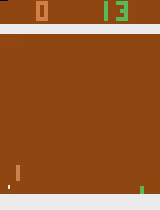## RL Experiments

Reinforcement learning, DQN.

RL Experiments

I worked on this project to get some basic knowledge of reinforcement learning and have some fun solving problems automatically. All three problems in this project was solved using Q learning.

```                ```
Algorithm double_q_learning:
initialize Q_1 = Q_2, memory_deque = []
initialize explore_rate = 1, time_step = 0
initialize state
repeat:
action = select from (random_action, argmax(state, Q_1)) with explore_rate
new_state, reward, done = environment.step(action)
memory_deque.append( (state, action, new_state, reward, done) )
time_step += 1
explore_rate = max(explore_rate * explore_rate_decay, explore_rate_min)
if time_step % update_q2_steps == 0:
train_batch = sample(memory_deque)
q_val = bellman_qeuation(train_batch)
Q_2 = Q_2 + back_propagation(q_val)
if time_step % update_q1_steps == 0:
Q_1 = Q_2
until avg_game_reward > threshold
```
```I used the template code from the UCB RL course homework to solve the Atari games. It is a deep Q learning algorithm, with some best practice, including double Q networks. The agent constantly achieved a average reward of over 20 on Pong-v0 after 4.3M of game steps.

The cart-pole problem should be the easiest and most common one. Nothing mysterious here. It was solved in 456 steps, which is a pretty good (and lucky) score for q learning.

I also developed an apple-picker game environment, a easy problem to apply deep Q learning with convolutional neural network, to test the code implementation. The problem was solved relatively easy, and should be a good start point for complex problems.